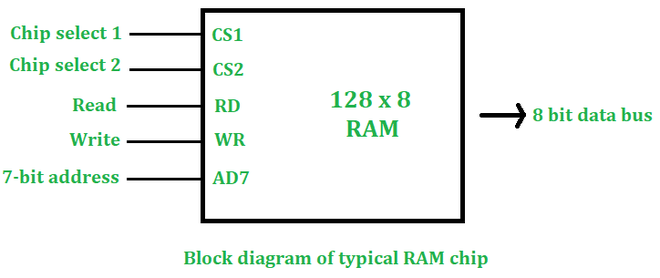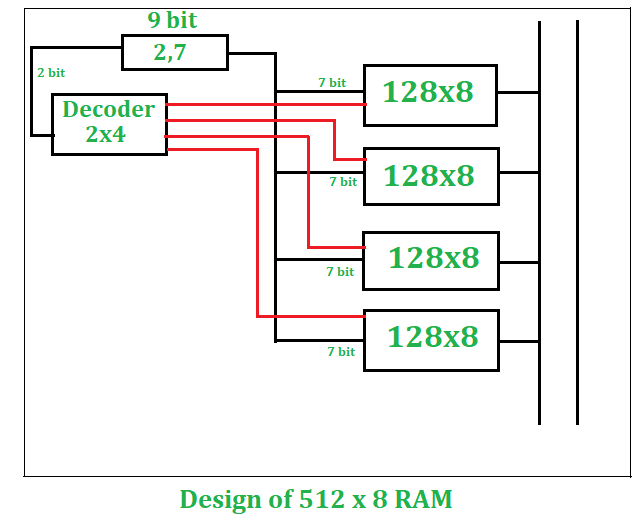# Design of 512×8 RAM using 128×8 RAM

• Difficulty Level : Expert
• Last Updated : 23 Feb, 2021

If we see RAM physically then we notice that RAM is made up of a few chips. We can design the required RAM size using basic RAM chips.

We can observe a basic RAM chip as follows: –1. CS1: –
For chip select 1, the value should be 1
2. CS2: –
For chip select 2, the value should be 0.
Used for upcoming signals.

If we have n bit address and m bit words then our RAM size will be 2n x m.

Example:

```n=7 bit, m=8 bit
RAM size= 128 x 8 ```

Given: –

```Basic RAM size = 128 x 8
Required RAM size  = 512 x 8```

To design a RAM size of 512×8 from 128×8, here are some calculations we need to do first –

1. Number of chips required:

```Number of chips required  = Desired RAM Size/ Basic RAM Size
=512x8/128x8
=4 chips```

```Required Size is 512 x 8
512 x 8= 29 x 8
Therefore, 9 bit address is required```

3. Decoder Size:
If the number of words is increasing them there will be a need for a decoder.
In this case number of words is increasing (from 128 to 512).

```Size of decoder= number of times words increasing
128⇢512
words increased by 4 times
Decoder Size= 2x4```

Pictorial Description (Design of 512×8 RAM):

From the above calculations, we see that we need four 128 x 8 RAM chips to design a 512 x 8 RAM size. If only the number of words is increasing then all chips will be arranged vertically. In this case, only the number of words is increasing (128-512) so all chips will be arranged vertically.

1. In a 512 x 8 RAM, there is a 9-bit address, we will divide this 9-bit address into 2 and 7-bit addresses.
2. The 2-bit address will be input as a 2 x 4 decoder, this decoder will have 4 output and the input will have 2 bits.
3. The 4 output of the decoder will enable every RAM of 128 x 8 individually.
4. Also, we can write 128 x 8 RAM chip as 27 x 8, every RAM chip will need a 7-bit address. We will connect the remaining 7-bit address line to every RAM.
5. This 7-bit address will select any word from the 128 words of that RAM, the selected word will be the output as the 8-bit word in the output bus.My Personal Notes arrow_drop_up